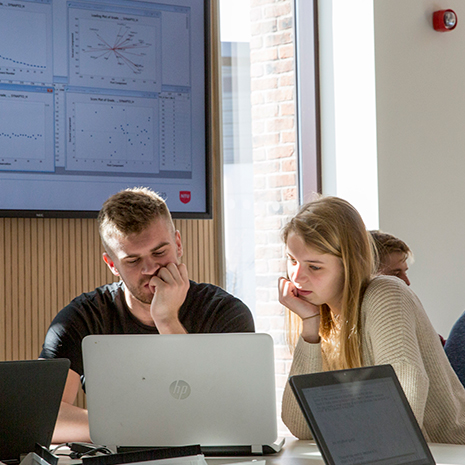# Introduction to Bayesian Data Analysis with R (online)

Info

## Course Information#### Course start date: Wednesday 21 June 2023 to Friday 23 June 2023

Duration: 3 day online course

Course Module: Non-accredited

On this three-day course, you will gain a solid introduction to Bayesian methods, both theoretically and practically.

We will teach the fundamental concepts of Bayesian inference and Bayesian modelling, including how Bayesian methods differ from their classical statistics counterparts, and show how to do Bayesian data analysis in practice in R.

This course is aimed at anyone who is interested to learn and apply Bayesian data analysis in any area of science, including the social sciences, life sciences, physical sciences.

No prior experience or familiarity with Bayesian statistics is required.

You can sign up for this course at any point within the live period until 3 working days prior to the course commencing.

#### Course Code

SSSIBDAR
Course Description

The course will cover these key topics:

• an overview of what Bayesian data analysis is, how it fits into practical data analysis and statistics, and how Bayesian approaches can be blended with traditional classical approaches to statistics
• introduction to Bayes’ rule and how they can be used as a means for performing statistical inference
• Bayesian analysis of normal linear regression models, which can be used to illustrate important and interesting parallels between Bayesian and classical or frequentist analyses, giving two different perspectives on the same problem
• application of Markov Chain Monte Carlo (MCMC) to Bayesian inference in practice using the acclaimed `brms` package in R
• Bayesian model comparison using cross-validation, information criteria, Bayes factors
• application of Bayesian methods to generalised linear models
• application of Bayesian methods to multilevel and mixed effects models

During the course you’ll:

• gain a solid introduction to Bayesian methods, both theoretically and practically and how they differ from their classical statistical counterparts, traditionally taught in statistics courses
• develop an understanding of the fundamental concepts of Bayesian inference and Bayesian modelling using R and the brms package, and how these differ from their classical statistics counterparts
• discover Bayesian approaches to linear regression, generalized linear models, and multilevel and mixed effects models
• learn how to use the brms package for Markov Chain Monte Carlo (MCMC) based inference

What will I gain?

By the end of the course, you’ll have learnt the fundamental principles of statistical inference using Bayes’s theorem and how this differs from classical statistical inference using frequentist sampling theory, and how the Bayesian methods compare to the frequentist counterparts.

You’ll also understand the general purpose of Monte Carlo algorithms for Bayesian inference in statistical models and how to apply Monte Carlo based Bayesian modelling software for model fitting and inference in a wide range of statistical models.

You’ll be able to evaluate Bayesian model fit using cross validation and information criteria, and compare different models of the same phenomena.

On completion of at least 80% of the course, you’ll receive a certificate of attendance.

Where you'll learn: The course is delivered through interactive online workshops via Zoom.

It will be practical, hands-on, and workshop based.

There will be some brief lecture style presentations throughout, i.e., using slides or blackboard, to introduce and explain key concepts and theories.

Throughout the course, and we will use real-world data sets and coding examples.

Tutor Profile: Mark Andrews is an Associate Professor at Nottingham Trent University whose research and teaching is focused on statistical methodology in research in the social and biological sciences.

He is the author of 2021 textbook on data science using R that is aimed at scientific researchers, and has a forthcoming new textbook on statistics and data science that is aimed at undergraduates in science courses.

His background is in computational cognitive science and mathematical psychology.

Any questions?  Contact [email protected], Commercial Manager, School of Social Sciences

Other available online CPD courses in this series include

Introduction to statistics using R and Rstudio

Introduction to Data Wrangling using R and tidyverse

Introduction to Data Visualization with R using ggplot

Introduction to Generalised Linear Models in R

Introduction to Multilevel (hierarchical, or mixed effects) Models in R

StartEndCourse Fee